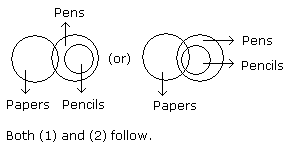# Verbal Reasoning - Syllogism

Exercise : Syllogism - Syllogism 1
Directions to Solve

In each of the following questions two statements are given and these statements are followed by two conclusions numbered (1) and (2). You have to take the given two statements to be true even if they seem to be at variance from commonly known facts. Read the conclusions and then decide which of the given conclusions logically follows from the two given statements, disregarding commonly known facts.

• (A) If only (1) conclusion follows
• (B) If only (2) conclusion follows
• (C) If either (1) or (2) follows
• (D) If neither (1) nor (2) follows and
• (E) If both (1) and (2) follow.

1.

Statements: Some actors are singers. All the singers are dancers.

Conclusions:

1. Some actors are dancers.
2. No singer is actor.

Only (1) conclusion follows
Only (2) conclusion follows
Either (1) or (2) follows
Neither (1) nor (2) follows
Both (1) and (2) follow
Explanation: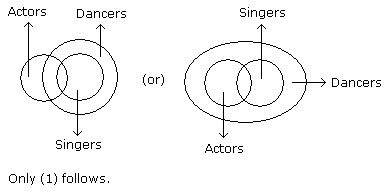2.

Statements: All the harmoniums are instruments. All the instruments are flutes.

Conclusions:

1. All the flutes are instruments.
2. All the harmoniums are flutes.

Only (1) conclusion follows
Only (2) conclusion follows
Either (1) or (2) follows
Neither (1) nor (2) follows
Both (1) and (2) follow
Explanation: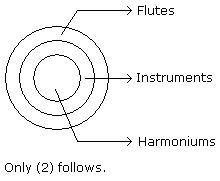3.

Statements: Some mangoes are yellow. Some tixo are mangoes.

Conclusions:

1. Some mangoes are green.
2. Tixo is a yellow.

Only (1) conclusion follows
Only (2) conclusion follows
Either (1) or (2) follows
Neither (1) nor (2) follows
Both (1) and (2) follow
Explanation: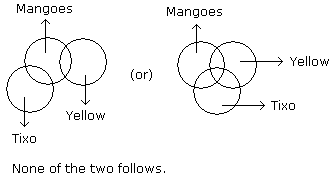4.

Statements: Some ants are parrots. All the parrots are apples.

Conclusions:

1. All the apples are parrots.
2. Some ants are apples.

Only (1) conclusion follows
Only (2) conclusion follows
Either (1) or (2) follows
Neither (1) nor (2) follows
Both (1) and (2) follow
Explanation: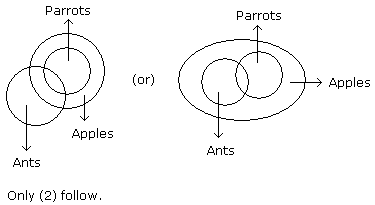5.

Statements: Some papers are pens. All the pencils are pens.

Conclusions:

1. Some pens are pencils.
2. Some pens are papers.

Only (1) conclusion follows
Only (2) conclusion follows
Either (1) or (2) follows
Neither (1) nor (2) follows
Both (1) and (2) follow